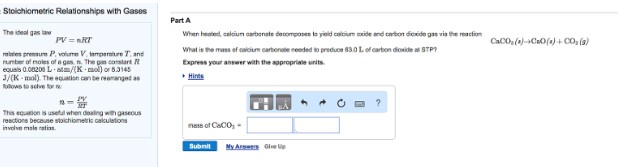# Problem: The ideal gas law PV = nRT relates pressure P, volume V, temperature T, and number of moles of a gas, n. The gas constant R equals 0.08208 L middot atm/(K • mol) or 8.3145 J/(K • mol). The equation can be rearranged as follows to for n: n = PV/RT This equation is useful when dealing with gaseous because calculations involve mole ratios. Part AWhen heated, calcium carbonate decomposes to yield calcium oxide and carbon dioxide gas via the reaction CaCO3  → CaO + CO2  What is the mass of calcium carbonate needed to produce 63.0 L of carbon dioxide at STP? Express your answer with the appropriate units.

###### FREE Expert Solution
99% (431 ratings)###### Problem Details

The ideal gas law

PV = nRT

relates pressure P, volume V, temperature T, and number of moles of a gas, n. The gas constant R equals 0.08208 L middot atm/(K • mol) or 8.3145 J/(K • mol). The equation can be rearranged as follows to for n:

n = PV/RT

This equation is useful when dealing with gaseous because calculations involve mole ratios.

Part A

When heated, calcium carbonate decomposes to yield calcium oxide and carbon dioxide gas via the reaction

CaCO3  → CaO + CO2

What is the mass of calcium carbonate needed to produce 63.0 L of carbon dioxide at STP?